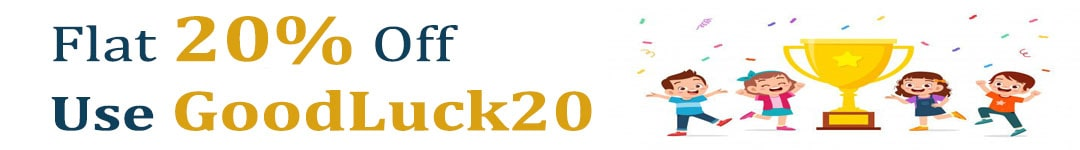International Maths Olympiad Forum By SOF Olympiad Trainer - Page 437

# User ForumSubject :IMO    Class : Class 9

## Ans 1: (Master Answer)

Class : Class 1

The correct answer is B.## Ans 2: (Master Answer)

Class : Class 1

The correct answer is B.Subject :IMO    Class : Class 5

## Ans 1:

Class : Class 6
correct answer is B

## Ans 2: (Master Answer)

Class : Class 1

The correct answer is C.

Perimeter of each square = 32 cm
⇒ 4 × side of square = 32 cm
⇒ Side of square = (32 ÷ 4) cm = 8 cm
∴ Perimeter of the figure = 10 × 8 = 80 cm

## Ans 3:

Class : Class 8
What a stupid master!

Subject :IMO    Class : Class 5

Class : Class 7

Class : Class 5

Class : Class 5
NO HARISH

## Ans 4:

Class : Class 10
the answer can be 'c' as there is not enough info on 'r'

## Ans 5:

Class : Class 7
There is no correct info about N .

## Ans 6:

Class : Class 9
I also know that the correct answer is A

Class : Class 7

## Ans 8: (Master Answer)

Class : Class 1

The correct answer is A.

The order from eldest to youngest is
M, N or R, Q
So, M is the eldest.

## Ans 9:

Class : Class 5
I agree with ARUNAV

## Ans 10:

Class : Class 8
the data is inadequate becuse n can be greater or smaller than m because it is mentioned only that n is not as old as m and also it is not mentioned relationship between r ad n

Subject :IMO    Class : Class 9

## Ans 1: (Master Answer)

Class : Class 1

The answer is BSubject :IMO    Class : Class 4

## Ans 1: (Master Answer)

Class : Class 1

The correct answer is B.

Quantity of flour Beena had = 4 kg = 4000 g
Quantity of flour used to bake cake = 3 kg 60 g = 3060 g
Quantity of flour left with Beena = 4000 – 3060 = 940 g
Hence, the right answer is Option B.

## Ans 2:

Class : Class 4
Quantity of flour Beena had =4 kg = 4000 g. Quantity of flour used to bake cake = 3 kg 60 g = 3060 gQuantity of flour left with Beena = 4000 -3060 = 940 gHence the correct answer is Option B

## Ans 3:

Class : Class 4

Subject :IMO    Class : Class 6

## Ans 1:

Class : Class 7
A is cooler than B

Class : Class 8

Class : Class 8
a

Class : Class 9
a

## Ans 5:

Class : Class 7
since, (-4) lies left to (-1) on a number line.so, -4< -1.: A is cooler than B

## Ans 6:

Class : Class 6
Option a. A is cooler than B because if you draw a number line,-4 is less than -1. When we go to the left it is decreasing and when it goes to right, it is ascending. Regards, class 6 boy Can't tell my name.

Subject :IMO    Class : Class 6

Class : Class 9
c

Class : Class 6
EASY

## Ans 3:

Class : Class 6
If we take a as 4 and b as 2, then according to communicative property, 4-2=2 but 2-4=-2 so 2 and -2 are not equal but, if we take a as 4 and b as 4, then it will be 4-4=0 and 4-4=0. Thus, a should be = b

Subject :IMO    Class : Class 6

## Ans 1:

Class : Class 9
THE ANSWER SHOULD BE 81 BECAUSE,SQUARE OF 9=9*9[NINE MULTIPLY BY NINE] HENCE THE ANSWER IS EIGHTY ONE.

Class : Class 5

Class : Class 5

## Ans 4:

Class : Class 5

Subject :IMO    Class : Class 3

Class : Class 3
B

Class : Class 6

Class : Class 5
D

Class : Class 3
D

Class : Class 4

Class : Class 6

Class : Class 5
D